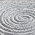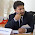Friday, November 18, 2011

How to convert a table into single column or row in Excel

In order to convert the table to a single column do the following. The data table has been assigned the name DataTable and the column name is ColumnData. It is sufficient to name only the first cell. Enter the following formula in the first cell of ColumnData and fill down as many rows as there are elements in your table:

=OFFSET(DataTable,MOD(ROW()-ROW(ColumnData),ROWS(DataTable)),TRUNC((ROW()-ROW(ColumnData))/ROWS(DataTable),0),1,1)

This will fill in the values from the DataTable in column-by-column order, working down then across. You can retrieve the values from DataTable in row-by-row order order, working across then down, with the following formula:

=OFFSET(DataTable,TRUNC((ROW()-ROW(ColumnData))/COLUMNS(DataTable),0),MOD(ROW()-ROW(ColumnData),COLUMNS(DataTable)),1,1)

The principle is the same: Enter the following formula in the first cell of ColumnData and fill down as many rows as there are elements in your table.

1.my data is in a 12 column by 8 row table whose upper left cell is in d7.

i would like to copy the table into a single row starting at ad7 and proceeding 96 cells to the right.

can you help?

2.Thanks, just what I needed :)

3.Thank you very much. This saved me a lot of time.

4.does this work on excel on mac? I'm asking cuz i tried it a lot and its not working on my excel on mac :(

5.Awesome! Worked well. Thank you

6.Well Done! - Biotech in S.S.F.

7.Remember to use the F9 key after pasting in new values